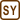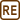## Blowing the Light Bulb

### Task number: 1041

Two 24 V light bulbs have power ratings of 25 W and 150 W. They are connected in series to a 230-volt electricity network. Determine which of the light bulbs will blow.

• #### Hint 1

Recall or look up what the term power rating of a light bulb means. How is the power rating related to other characteristics of the light bulb?

• #### Hint 2

Realize what equations hold for the overall voltage and electric current that flows in the circuit where the light bulbs are connected in series.

• #### Analysis

The main aim of this task is to calculate the real voltage across both light bulbs connected in the electricity network. Then, these voltages will be compared with the maximal allowed voltage stated on the light bulbs (the nominal voltage). If the real voltage across one of the light bulbs is higher than the nominal value, then the light bulb filament will most likely burn.

First, we can determine the electric resistance of both light bulbs from the stated nominal values and power ratings. The electric resistance is a characteristic that does not depend on the way how the light bulb is connected in the circuit. For this reason, the electric resistance will be the same in all connections.

The light bulbs are connected in series, therefore the currents flowing through the light bulbs will be equal. Thus, it follows from the Ohm’s law that the ratio of the electric resistance of both light bulbs is equal to the ratio of the real voltages across the light bulbs. The sum of the voltages across the light bulbs has to be equal to the voltage of the electric source. Now, we determine the voltages across both light bulbs from these two equalities, and we compare them with the nominal values that are stated on the light bulbs.

• #### Solution – expression of the voltages across the light bulbs

Firstly, we determine the electric resistances R1 and R2 of the light bulbs by using their power ratings. The power rating P1 and P2 of the light bulbs can be calculated from the formulas:

$P_1\,=\,\frac{U^2}{R_1}\hspace{15px}\mathrm{a}\hspace{15px}P_2\,=\,\frac{U^2}{R_2}\hspace{10px}\mathrm{,}$

where U means maximal allowed voltage of the light bulbs (the nominal voltage) that is 24 V in this task. Therefore, the ratio of the power ratings is equal to the inverse ratio of the light bulbs electric resistances:

$\frac{P_1}{P_2}\,=\,\frac{\frac{U^2}{R_1}}{\frac{U^2}{R_2}}\,=\,\frac{R_2}{R_1} \tag{(1)}$

The light bulbs are connected in series. For this reason, the amount of the currents flowing through both light bulbs is the same. Thus, this equation follows from the Ohm’s law:

$I\,=\,\frac{U_1}{R_1}\,=\,\frac{U_2}{R_2}\,,$

U1 and U2 are the real voltages across the light bulbs.

And thus:

$\frac{R_2}{R_1}\,=\,\frac{U_2}{U_1}$

Now, we substitute this equation into the formula (1):

$\frac{P_1}{P_2}\,=\,\frac{R_2}{R_1}\,=\,\frac{U_2}{U_1}$

The sum of the voltages U1 and U2 across the light bulbs is equal to the voltage of the electric source Uel in series connection. So it holds:

$U_{el}\,=\,U_1\,+\,U_2$

We express the voltage U2 from this equation and substitute it into the expression of the ratio of the power rating:

$U_2\,=\,U_{el}\,-\,U_1$ $\frac{P_1}{P_2}\,=\,\frac{U_2}{U_1}\,=\,\frac{U_{el}\,-\,U_1}{U_1}$

Now, we can calculate the real voltage across the first light bulb depending on the power ratings of both light bulbs and the overall voltage of the electricity network:

$\frac{P_1}{P_2}\,U_1\,=\,U_{el}\,-\,U_1$ $\left(\frac{P_1}{P_2}\,+\,1\right)\,U_1\,=\,U_{el}$ $\frac{P_1\,+\,P_2}{P_2}\,U_1\,=\,U_{el}$

The voltage across the first light bulb can be calculated from the equation:

$U_1\,=\,\frac{P_2}{P_1\,+\,P_2}\,U_{el}$

We use similar method to calculate the real voltage across the second light bulb. However, only two light bulbs are connected in the circuit and there is no other component to influence them. For this reason, it is sufficient to interchange the subscripts of the voltage and the power ratings in the gained equation (the subscript 1 will be substitute for the subscript 2 and vice versa).

The equation for calculation of the voltage across the second light bulb is:

$U_2\,=\,\frac{P_1}{P_1\,+\,P_2}\,U_{el}$
• #### Numerical Values

We know from the task assignment:

 Uel = 230 V overall voltage of the electricity network P1 = 25 W power rating of the first light bulb P2 = 150 W power rating of the second light bulb U = 24 V maximal allowed voltage across the light bulbs

We gained equations for calculation of the voltages across both light bulbs in the last section:

$U_1\,=\,\frac{P_2}{P_1\,+\,P_2}\,U_{el}$ $U_2\,=\,\frac{P_1}{P_1\,+\,P_2}\,U_{el}$

Now, we can replace the physical quantities with the assigned numerical values:

$U_1\,=\,\frac{150\,\mathrm{W}}{25\,\mathrm{W}\,+\,150\,\mathrm{W}}\,\cdot\,230\,\mathrm{V}\,\dot{=}\,200\,\mathrm{V}$ $U_2\,=\,\frac{25\,\mathrm{W}}{25\,\mathrm{W}\,+\,150\,\mathrm{W}}\,\cdot\,230\,\mathrm{V}\,\dot{=}\,33\,\mathrm{V}$
• #### Answer and discussion of the results

The real voltage across the light bulb with a power rating of 25 W is almost 200 V and the real voltage across the light bulb with a power rating of 150 W is 33 V. The maximal allowed voltage is exceeded in both cases. Nevertheless, the overload is much higher in the case of the light bulb with the lower power rating, and therefore this light bulb would probably blow first. This would disconnect the electric circuit and the second light bulb – where the allowed voltage is exceeded narrowly – would be saved.